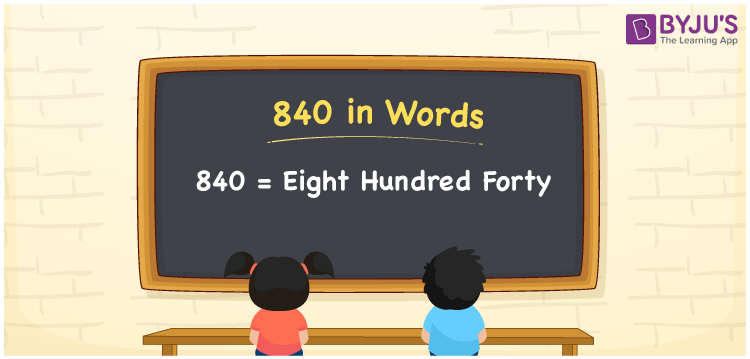# 840 in Words

840 in words is written as Eight hundred forty. In both the International System of Numerals and the Indian System of Numerals, 840 is written as Eight hundred forty. The number 840 is a Cardinal Number as it represents some quantity. For example, “the total cost of all these products is rupees 840”.

 840 in Words Eight hundred forty Eight hundred forty in Number 840

## 840 in English Words

We write 840 in English Words using the letters of the English alphabet. Therefore, we read 840 in English as “Eight hundred forty.”## How to Write 840 in Words?

To write 840 in words, we shall use the place value chart. In the place value chart, write 0 in the ones, 4 in the tens, and 8 in the hundreds. Now let us make a place value chart to write the number 840 in words.

 Hundreds Tens Ones 8 4 0

Thus, we can write the expanded form as

8 × Hundred + 4 × Ten + 0 × One

= 8 × 100 + 4 × 10 + 0 × 1

= 800 + 40 + 0

= 840

= Eight Hundred Forty

840 is a natural number which is the successor of 839 and the predecessor of 841.

840 in words – Eight Hundred Forty

• Is 840 an odd number? – No
• Is 840 an even number? – Yes
• Is 840 a perfect square number? – No
• Is 840 a perfect cube number? – No
• Is 840 a prime number? – No
• Is 840 a composite number? – Yes

## Frequently Asked Questions on 840 in Words

Q1

### How to write 840 in words?

840 in words is written as Eight Hundred Forty.
Q2

### How to write 840 in words in the International and Indian System of Numerals?

In both the system of numerals, 840 is written as Eight Hundred Forty.
Q3

### How to write 840 in a place value chart?

In the place value chart, write 0 in the ones, 4 in the tens, and 8 in the hundreds.